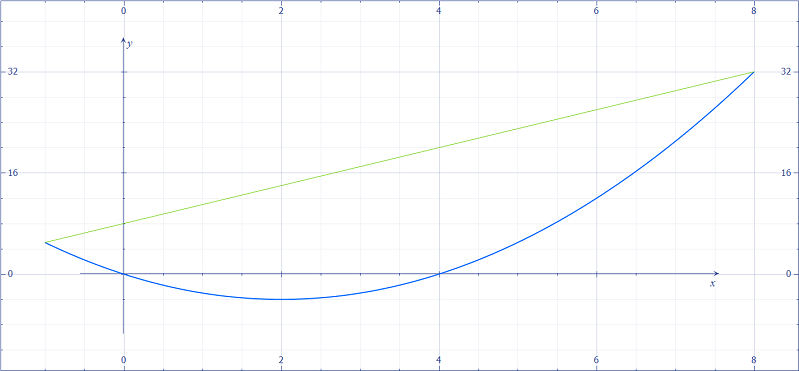# Find the area bounded by y=x^2 - 4x and y= 3x+8.

## Question:

Find the area bounded by {eq}\displaystyle y=x^{2}-4x \ and \ y=3x+8 {/eq}

## Area Between Curves

The area between two curves {eq}f(x) {/eq} and {eq}g(x) {/eq} between {eq}x=a {/eq} and {eq}x=b {/eq} with {eq}f(x)\geq g(x) \text{ for all } x\in [a,b]. {/eq} can be found by evaluating

{eq}\displaystyle \int_a^b (f(x)-g(x))\, dx {/eq}

Below is the graph of {eq}y=x^2-4x {/eq} and {eq}y=3x+8. {/eq}Note that {eq}y=3x+8 {/eq} and the curves intersect when

{eq}3x+8=x^2-4x\\ x...

Become a Study.com member to unlock this answer! Create your account